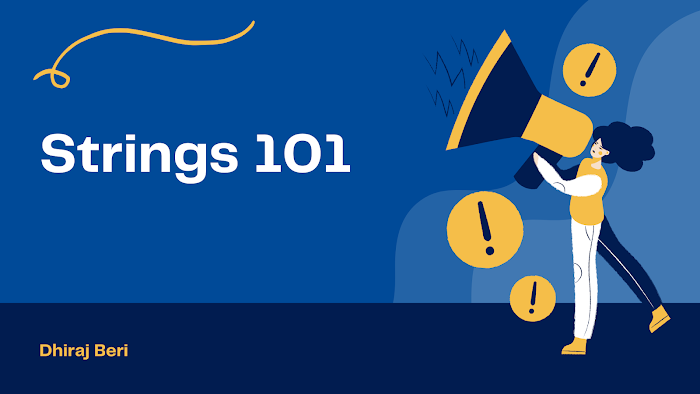# Strings Operations

Length of string
`#include <stdio.h>int main(){    char s[] = "Dhiraj";    int i=0;    while(s[i] != '\0')    {        i++;    }    printf("length of string is %d", i);    return 0;}`

Changing Case
`#include <stdio.h>int main(){    char s[] = "dhiraj";    int i=0;    while(s[i] != '\0')    {        if(s[i] >= 65 && s[i] <=90)            s[i] = s[i] + 32;        else            s[i] = s[i] - 32;        i++;    }    printf("%s", s);    return 0;}`

Counting number of vowels
`#include <stdio.h>int main(){    char a[] = "aeiissou";    int i, count=0;    for(i=0; a[i]!='\0';i++)    {        if(a[i]=='a' || a[i]=='e' || a[i]=='i' || a[i]=='o' || a[i]=='u')        {            count++;        }    }    printf("%d", count);    return 0;}`

Number of words in a string
`#include <stdio.h>int main(){    char a[] = "dhiraj beri";    int i, word=1;    for(i=0; a[i]!='\0';i++)    {        if(a[i]==' ' && a[i-1]!=' ');//white space condition        {            word++;        }    }    printf("%d", word);    return 0;}`

Validate a string
`#include <stdio.h>int main(){    char a[] = "[email protected]";    int i;    for(i=0; a[i]!='\0';i++)    {        if(!(a[i]>=65 && a[i]<=90) && !(a[i]>=97 && a[i]<=122))        {            return 0;        }    }    return 1;}`

Reversing the string
`#include <stdio.h>int main(){    char a[] = "python";    int i, j;    for(j=0;a[j]!='\0';j++){}    j=j-1;    for(i=0;i<j;i++,j--)    {        char t;        t=a[i];        a[i]=a[j];        a[j]=t;    }    printf("%s", a);    return 0;}`

Comparing strings and palindrome
`#include <stdio.h>int main(){    char a[] = "python";    char b[] = "python";    int i,j;    for(i=0,j=0; a[i]!='\0', b[j]!='\0'; i++,j++)    {        if(a[i]!=b[j])        {            return 0; //not same or not palindrome        }    }    return 1; //same or palindrome}`

Finding the duplicates in a string
`// 1. Compare with other elements// 2. Using hashtable or counting// 3. Using Bits// Biwise Opereations// 1. Left shift <<// 2. Bits ORing (Merging)// 3. BIts ANDing (Masking)`

Check for Anagram
`#include <stdio.h>int main(){    char s1[] = "decimal";    char s2[] = "medical";    // sbse pehle ascii code nikalo    // for example d=100, 100-97=3, increment position 3 in hash mutable    // scan through second string, and for each element, for example m=109, 109-97=12, goto index 12 and decrement it    // If we not got -1 value in hash table then this both strings are anagram otherwise not anagram    // first string ko linear (n), second string ko linear (n), so the time complexity is O(n)    int i, a, b, H={0};    for(i=0; s1[i]!='\0'; i++){}    a = i;  //where a is length of string 1    for(i=0; s2[i]!='\0'; i++){}    b = i;  //where b is length of string 2    // If both string length is same they are anagram otherwise they are not anagram    if (a!=b)    {        printf("Not anagram");    }    else    {        for(i=0; s1[i]!='\0'; i++)        {            H[s1[i]-97]++;        }        for(i=0; s2[i]!='\0'; i++)        {            H[s2[i]-97]--;            if (H[s2[i]-97]<0)            {                printf("Not an Anagram");                break;            }        }        if(s2[i]=='\0')        {            printf("Anagram");        }    }    return 0;}`

State-space tree Bruteforce Backtracking

Whenever we have to go back and take another route we can implement it by recursion.

We have to use recursion to achieve backtracking and with the help of backtracking, we perform brute force.

Permutations of a string
`// means all possible arrangement of string// s = ABC then n! possible, 3! = 6 possible arrangement// Using swapping#include <stdio.h>void perm(char s[], int l, int h){    int i;    if (l == h)    {        printf("%s\n", s);    }    for (i = l; i <= h; i++)    {        swap(s[l], s[i]);        perm(s, l + 1, h);        swap(s[l], s[i]);    }}int main(){    char s[] = "ABC";    perm(s, 0, 2);    return 0;}`

Congratulations to you, if you really understand this section. Any doubts? Then you can ask me in the comment section.

As we covered strings, this is the right time to solve some Leetcode easy strings questions.

What's next?

After practicing, you are ready for the next data structure - Linked List.